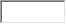• FAQ
• Contact/ Homework Answers / Physics / Question What the equivalent resistance between points a and b in
Not my Question
Flag Content

# Question : Question What the equivalent resistance between points a and b in

Question

What is the equivalent resistance between points a and b in the figure below? In the figure, R1 = 51 ? and R2 = 39 ?.

1?

## Solution 5 (1 Ratings )

Solved
Physics 4 Months Ago 2 Views